Precalculus 2e

# 8.1Non-right Triangles: Law of Sines

Precalculus 2e8.1 Non-right Triangles: Law of Sines

### Learning Objectives

In this section, you will:

• Use the Law of Sines to solve oblique triangles.
• Find the area of an oblique triangle using the sine function.
• Solve applied problems using the Law of Sines.

To ensure the safety of over 5,000 U.S. aircraft flying simultaneously during peak times, air traffic controllers monitor and communicate with them after receiving data from the robust radar beacon system. Suppose two radar stations located 20 miles apart each detect an aircraft between them. The angle of elevation measured by the first station is 35 degrees, whereas the angle of elevation measured by the second station is 15 degrees. How can we determine the altitude of the aircraft? We see in Figure 1 that the triangle formed by the aircraft and the two stations is not a right triangle, so we cannot use what we know about right triangles. In this section, we will find out how to solve problems involving non-right triangles.

Figure 1

### Using the Law of Sines to Solve Oblique Triangles

In any triangle, we can draw an altitude, a perpendicular line from one vertex to the opposite side, forming two right triangles. It would be preferable, however, to have methods that we can apply directly to non-right triangles without first having to create right triangles.

Any triangle that is not a right triangle is an oblique triangle. Solving an oblique triangle means finding the measurements of all three angles and all three sides. To do so, we need to start with at least three of these values, including at least one of the sides. We will investigate three possible oblique triangle problem situations:

1. ASA (angle-side-angle) We know the measurements of two angles and the included side. See Figure 2.
Figure 2
2. AAS (angle-angle-side) We know the measurements of two angles and a side that is not between the known angles. See Figure 3.
Figure 3
3. SSA (side-side-angle) We know the measurements of two sides and an angle that is not between the known sides. See Figure 4.
Figure 4

Knowing how to approach each of these situations enables us to solve oblique triangles without having to drop a perpendicular to form two right triangles. Instead, we can use the fact that the ratio of the measurement of one of the angles to the length of its opposite side will be equal to the other two ratios of angle measure to opposite side. Let’s see how this statement is derived by considering the triangle shown in Figure 5.

Figure 5

Using the right triangle relationships, we know that $sinα= h b sinα= h b$ and $sinβ= h a . sinβ= h a .$ Solving both equations for $h h$ gives two different expressions for $h. h.$

$h=bsinαandh=asinβ h=bsinαandh=asinβ$

We then set the expressions equal to each other.

Similarly, we can compare the other ratios.

$sinα a = sinγ c and sinβ b = sinγ c sinα a = sinγ c and sinβ b = sinγ c$

Collectively, these relationships are called the Law of Sines.

$sinα a = sinβ b = sinγ c sinα a = sinβ b = sinγ c$

Note the standard way of labeling triangles: angle $α α$ (alpha) is opposite side $a; a;$ angle $β β$ (beta) is opposite side $b; b;$ and angle $γ γ$ (gamma) is opposite side $c. c.$ See Figure 6.

While calculating angles and sides, be sure to carry the exact values through to the final answer. Generally, final answers are rounded to the nearest tenth, unless otherwise specified.

Figure 6

### Law of Sines

Given a triangle with angles and opposite sides labeled as in Figure 6, the ratio of the measurement of an angle to the length of its opposite side will be equal to the other two ratios of angle measure to opposite side. All proportions will be equal. The Law of Sines is based on proportions and is presented symbolically two ways.

$sinα a = sinβ b = sinγ c sinα a = sinβ b = sinγ c$
$a sinα = b sinβ = c sinγ a sinα = b sinβ = c sinγ$

To solve an oblique triangle, use any pair of applicable ratios.

### Example 1

#### Solving for Two Unknown Sides and Angle of an AAS Triangle

Solve the triangle shown in Figure 7 to the nearest tenth.

Figure 7

### Try It #1

Solve the triangle shown in Figure 8 to the nearest tenth.

Figure 8

### Using The Law of Sines to Solve SSA Triangles

We can use the Law of Sines to solve any oblique triangle, but some solutions may not be straightforward. In some cases, more than one triangle may satisfy the given criteria, which we describe as an ambiguous case. Triangles classified as SSA, those in which we know the lengths of two sides and the measurement of the angle opposite one of the given sides, may result in one or two solutions, or even no solution.

### Possible Outcomes for SSA Triangles

Oblique triangles in the category SSA may have four different outcomes. Figure 9 illustrates the solutions with the known sides $a a$ and $b b$ and known angle $α. α.$

Figure 9

### Example 2

#### Solving an Oblique SSA Triangle

Solve the triangle in Figure 10 for the missing side and find the missing angle measures to the nearest tenth.

Figure 10

### Try It #2

Given $α=80°,a=120, α=80°,a=120,$ and $b=121, b=121,$ find the missing side and angles. If there is more than one possible solution, show both.

### Example 3

#### Solving for the Unknown Sides and Angles of a SSA Triangle

In the triangle shown in Figure 13, solve for the unknown side and angles. Round your answers to the nearest tenth.

Figure 13

### Try It #3

Given $α=80°,a=100,b=10, α=80°,a=100,b=10,$ find the missing side and angles. If there is more than one possible solution, show both. Round your answers to the nearest tenth.

### Example 4

#### Finding the Triangles That Meet the Given Criteria

Find all possible triangles if one side has length 4 opposite an angle of 50°, and a second side has length 10.

### Try It #4

Determine the number of triangles possible given $a=31, a=31,$ $b=26,b=26,$ $β=48°. β=48°.$

### Finding the Area of an Oblique Triangle Using the Sine Function

Now that we can solve a triangle for missing values, we can use some of those values and the sine function to find the area of an oblique triangle. Recall that the area formula for a triangle is given as $Area= 1 2 bh, Area= 1 2 bh,$ where $b b$ is base and $h h$ is height. For oblique triangles, we must find $h h$ before we can use the area formula. Observing the two triangles in Figure 15, one acute and one obtuse, we can drop a perpendicular to represent the height and then apply the trigonometric property $sinα= opposite hypotenuse sinα= opposite hypotenuse$ to write an equation for area in oblique triangles. In the acute triangle, we have $sinα= h c sinα= h c$ or $csinα=h. csinα=h.$ However, in the obtuse triangle, we drop the perpendicular outside the triangle and extend the base $b b$ to form a right triangle. The angle used in calculation is $α ′ , α ′ ,$ or $180−α. 180−α.$

Figure 15

Thus,

$Area= 1 2 ( base )( height )= 1 2 b( csinα ) Area= 1 2 ( base )( height )= 1 2 b( csinα )$

Similarly,

$Area= 1 2 a( bsinγ )= 1 2 a( csinβ ) Area= 1 2 a( bsinγ )= 1 2 a( csinβ )$

### Area of an Oblique Triangle

The formula for the area of an oblique triangle is given by

$Area= 1 2 bcsinα = 1 2 acsinβ = 1 2 absinγ Area= 1 2 bcsinα = 1 2 acsinβ = 1 2 absinγ$

This is equivalent to one-half of the product of two sides and the sine of their included angle.

### Example 5

#### Finding the Area of an Oblique Triangle

Find the area of a triangle with sides $a=90,b=52, a=90,b=52,$ and angle $γ=102°. γ=102°.$ Round the area to the nearest integer.

### Try It #5

Find the area of the triangle given $β=42°, β=42°,$ $a=7.2ft,a=7.2ft,$ $c=3.4ft. c=3.4ft.$ Round the area to the nearest tenth.

### Solving Applied Problems Using the Law of Sines

The more we study trigonometric applications, the more we discover that the applications are countless. Some are flat, diagram-type situations, but many applications in calculus, engineering, and physics involve three dimensions and motion.

### Example 6

#### Finding an Altitude

Find the altitude of the aircraft in the problem introduced at the beginning of this section, shown in Figure 16. Round the altitude to the nearest tenth of a mile.

Figure 16

### Try It #6

The diagram shown in Figure 17 represents the height of a blimp flying over a football stadium. Find the height of the blimp if the angle of elevation at the southern end zone, point A, is 70°, the angle of elevation from the northern end zone, point $B, B,$ is 62°, and the distance between the viewing points of the two end zones is 145 yards.

Figure 17

### Media

Access these online resources for additional instruction and practice with trigonometric applications.

### 8.1 Section Exercises

#### Verbal

1.

Describe the altitude of a triangle.

2.

Compare right triangles and oblique triangles.

3.

When can you use the Law of Sines to find a missing angle?

4.

In the Law of Sines, what is the relationship between the angle in the numerator and the side in the denominator?

5.

What type of triangle results in an ambiguous case?

#### Algebraic

For the following exercises, assume $α α$ is opposite side $a,β a,β$ is opposite side $b, b,$ and $γ γ$ is opposite side $c. c.$ Solve each triangle, if possible. Round each answer to the nearest tenth.

6.

$α=43°,γ=69°,a=20 α=43°,γ=69°,a=20$

7.

$α=35°,γ=73°,c=20 α=35°,γ=73°,c=20$

8.

$α=60°, α=60°,$ $β=60°,β=60°,$ $γ=60° γ=60°$

9.

$a=4, a=4,$ $α= 60° ,α= 60° ,$ $β=100° β=100°$

10.

$b=10, b=10,$ $β=95°,γ= 30° β=95°,γ= 30°$

For the following exercises, use the Law of Sines to solve for the missing side for each oblique triangle. Round each answer to the nearest hundredth. Assume that angle $A A$ is opposite side $a, a,$ angle $B B$ is opposite side $b, b,$ and angle $C C$ is opposite side $c. c.$

11.

Find side $b b$ when $A=37°, A=37°,$ $B=49°,B=49°,$ $c=5. c=5.$

12.

Find side $a a$ when $A=132°,C=23°,b=10. A=132°,C=23°,b=10.$

13.

Find side $c c$ when $B=37°,C=21°, B=37°,C=21°,$ $b=23. b=23.$

For the following exercises, assume $α α$ is opposite side $a,β a,β$ is opposite side $b, b,$ and $γ γ$ is opposite side $c. c.$ Determine whether there is no triangle, one triangle, or two triangles. Then solve each triangle, if possible. Round each answer to the nearest tenth.

14.

$α=119°,a=14,b=26 α=119°,a=14,b=26$

15.

$γ=113°,b=10,c=32 γ=113°,b=10,c=32$

16.

$b=3.5, b=3.5,$ $c=5.3,c=5.3,$ $γ= 80° γ= 80°$

17.

$a=12, a=12,$ $c=17,c=17,$ $α= 35° α= 35°$

18.

$a=20.5, a=20.5,$ $b=35.0,b=35.0,$ $β= 25° β= 25°$

19.

$a=7, a=7,$ $c=9,c=9,$ $α=43° α=43°$

20.

$a=7,b=3,β=24° a=7,b=3,β=24°$

21.

$b=13,c=5,γ=10° b=13,c=5,γ=10°$

22.

$a=2.3,c=1.8,γ=28° a=2.3,c=1.8,γ=28°$

23.

$β=119°,b=8.2,a=11.3 β=119°,b=8.2,a=11.3$

For the following exercises, use the Law of Sines to solve, if possible, the missing side or angle for each triangle or triangles in the ambiguous case. Round each answer to the nearest tenth.

24.

Find angle $A A$ when $a=24,b=5,B=22°. a=24,b=5,B=22°.$

25.

Find angle $A A$ when $a=13,b=6,B=20°. a=13,b=6,B=20°.$

26.

Find angle $B B$ when $A=12°,a=2,b=9. A=12°,a=2,b=9.$

For the following exercises, find the area of the triangle with the given measurements. Round each answer to the nearest tenth.

27.

$a=5,c=6,β= 35° a=5,c=6,β= 35°$

28.

$b=11,c=8,α= 28° b=11,c=8,α= 28°$

29.

$a=32,b=24,γ= 75° a=32,b=24,γ= 75°$

30.

$a=7.2,b=4.5,γ= 43° a=7.2,b=4.5,γ= 43°$

#### Graphical

For the following exercises, find the length of side $x. x.$ Round to the nearest tenth.

31.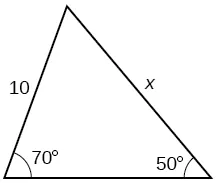32.33.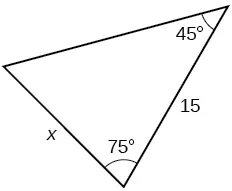34.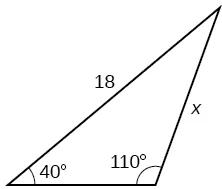35.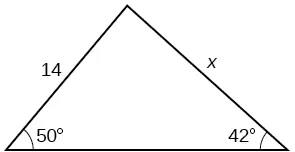36.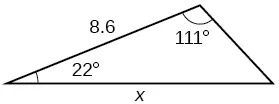For the following exercises, find the measure of angle $x, x,$ if possible. Round to the nearest tenth.

37.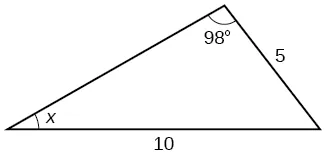38.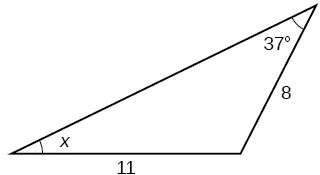39.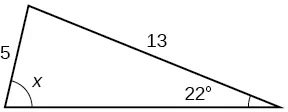40.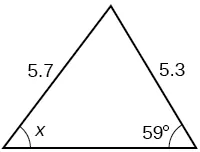41.

Notice that $x x$ is an obtuse angle.42.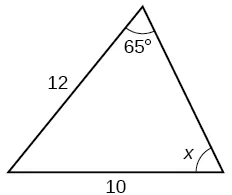For the following exercise, solve the triangle. Round each answer to the nearest tenth.

43.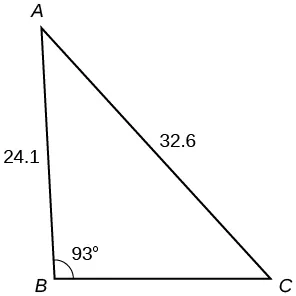44.

For the following exercises, find the area of each triangle. Round each answer to the nearest tenth.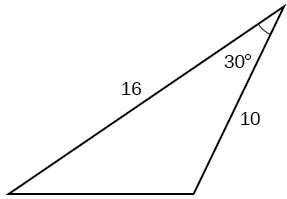45.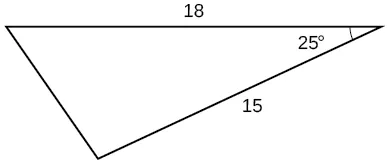46.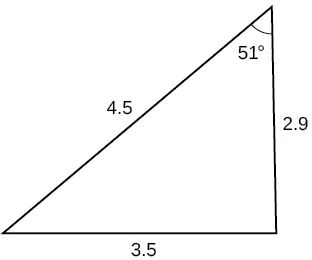47.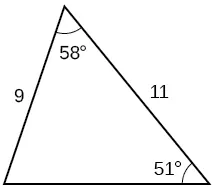48.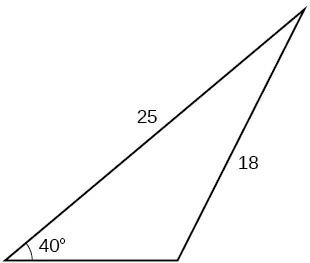49.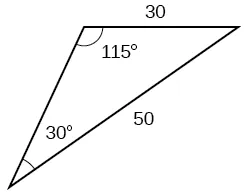#### Extensions

50.

Find the radius of the circle in Figure 18. Round to the nearest tenth.

Figure 18
51.

Find the diameter of the circle in Figure 19. Round to the nearest tenth.

Figure 19
52.

Find $m∠ADC m∠ADC$ in Figure 20. Round to the nearest tenth.

Figure 20
53.

Find $AD AD$ in Figure 21. Round to the nearest tenth.

Figure 21
54.

Solve both triangles in Figure 22. Round each answer to the nearest tenth.

Figure 22
55.

Find $AB AB$ in the parallelogram shown in Figure 23.

Figure 23
56.

Solve the triangle in Figure 24. (Hint: Draw a perpendicular from $H H$ to $JK). JK).$ Round each answer to the nearest tenth.

Figure 24
57.

Solve the triangle in Figure 25. (Hint: Draw a perpendicular from $N N$ to $LM). LM).$ Round each answer to the nearest tenth.

Figure 25
58.

In Figure 26, $ABCD ABCD$ is not a parallelogram. $∠m ∠m$ is obtuse. Solve both triangles. Round each answer to the nearest tenth.

Figure 26

#### Real-World Applications

59.

A pole leans away from the sun at an angle of $7° 7°$ to the vertical, as shown in Figure 27. When the elevation of the sun is $55°, 55°,$ the pole casts a shadow 42 feet long on the level ground. How long is the pole? Round the answer to the nearest tenth.

Figure 27
60.

To determine how far a boat is from shore, two radar stations 500 feet apart find the angles out to the boat, as shown in Figure 28. Determine the distance of the boat from station $A A$ and the distance of the boat from shore. Round your answers to the nearest whole foot.

Figure 28
61.

Figure 29 shows a satellite orbiting Earth. The satellite passes directly over two tracking stations $A A$ and $B, B,$ which are 69 miles apart. When the satellite is on one side of the two stations, the angles of elevation at $A A$ and $B B$ are measured to be $83.9° 83.9°$ and $86.2°, 86.2°,$ respectively. How far is the satellite from station $A A$ and how high is the satellite above the ground? Round answers to the nearest whole mile.

Figure 29
62.

A communications tower is located at the top of a steep hill, as shown in Figure 30. The angle of inclination of the hill is $67°. 67°.$ A guy wire is to be attached to the top of the tower and to the ground, 165 meters downhill from the base of the tower. The angle formed by the guy wire and the hill is $16°. 16°.$ Find the length of the cable required for the guy wire to the nearest whole meter.

Figure 30
63.

The roof of a house is at a $20° 20°$ angle. An 8-foot solar panel is to be mounted on the roof and should be angled $38° 38°$ relative to the horizontal for optimal results. (See Figure 31). How long does the vertical support holding up the back of the panel need to be? Round to the nearest tenth.

Figure 31
64.

Similar to an angle of elevation, an angle of depression is the acute angle formed by a horizontal line and an observer’s line of sight to an object below the horizontal. A pilot is flying over a straight highway. He determines the angles of depression to two mileposts, 6.6 km apart, to be $37° 37°$ and $44°, 44°,$ as shown in Figure 32. Find the distance of the plane from point $A A$ to the nearest tenth of a kilometer.

Figure 32
65.

A pilot is flying over a straight highway. He determines the angles of depression to two mileposts, 4.3 km apart, to be 32° and 56°, as shown in Figure 33. Find the distance of the plane from point $A A$ to the nearest tenth of a kilometer.

Figure 33
66.

In order to estimate the height of a building, two students stand at a certain distance from the building at street level. From this point, they find the angle of elevation from the street to the top of the building to be 39°. They then move 300 feet closer to the building and find the angle of elevation to be 50°. Assuming that the street is level, estimate the height of the building to the nearest foot.

67.

In order to estimate the height of a building, two students stand at a certain distance from the building at street level. From this point, they find the angle of elevation from the street to the top of the building to be 35°. They then move 250 feet closer to the building and find the angle of elevation to be 53°. Assuming that the street is level, estimate the height of the building to the nearest foot.

68.

Points $A A$ and $B B$ are on opposite sides of a lake. Point $C C$ is 97 meters from $A. A.$ The measure of angle $BAC BAC$ is determined to be 101°, and the measure of angle $ACB ACB$ is determined to be 53°. What is the distance from $A A$ to $B, B,$ rounded to the nearest whole meter?

69.

A man and a woman standing $3 1 2 3 1 2$ miles apart spot a hot air balloon at the same time. If the angle of elevation from the man to the balloon is 27°, and the angle of elevation from the woman to the balloon is 41°, find the altitude of the balloon to the nearest foot.

70.

Two search teams spot a stranded climber on a mountain. The first search team is 0.5 miles from the second search team, and both teams are at an altitude of 1 mile. The angle of elevation from the first search team to the stranded climber is 15°. The angle of elevation from the second search team to the climber is 22°. What is the altitude of the climber? Round to the nearest tenth of a mile.

71.

A street light is mounted on a pole. A 6-foot-tall man is standing on the street a short distance from the pole, casting a shadow. The angle of elevation from the tip of the man’s shadow to the top of his head of 28°. A 6-foot-tall woman is standing on the same street on the opposite side of the pole from the man. The angle of elevation from the tip of her shadow to the top of her head is 28°. If the man and woman are 20 feet apart, how far is the street light from the tip of the shadow of each person? Round the distance to the nearest tenth of a foot.

72.

Three cities, $A,B, A,B,$ and $C, C,$ are located so that city $A A$ is due east of city $B. B.$ If city $C C$ is located 35° west of north from city $B B$ and is 100 miles from city $A A$ and 70 miles from city $B, B,$ how far is city $A A$ from city $B? B?$ Round the distance to the nearest tenth of a mile.

73.

Two streets meet at an 80° angle. At the corner, a park is being built in the shape of a triangle. Find the area of the park if, along one road, the park measures 180 feet, and along the other road, the park measures 215 feet.

74.

Brian’s house is on a corner lot. Find the area of the front yard if the edges measure 40 and 56 feet, as shown in Figure 34.

Figure 34
75.

The Bermuda triangle is a region of the Atlantic Ocean that connects Bermuda, Florida, and Puerto Rico. Find the area of the Bermuda triangle if the distance from Florida to Bermuda is 1030 miles, the distance from Puerto Rico to Bermuda is 980 miles, and the angle created by the two distances is 62°.

76.

A yield sign measures 30 inches on all three sides. What is the area of the sign?

77.

Naomi bought a dining table whose top is in the shape of a triangle. Find the area of the table top if two of the sides measure 4 feet and 4.5 feet, and the smaller angles measure 32° and 42°, as shown in Figure 35.

Figure 35
Order a print copy

As an Amazon Associate we earn from qualifying purchases.